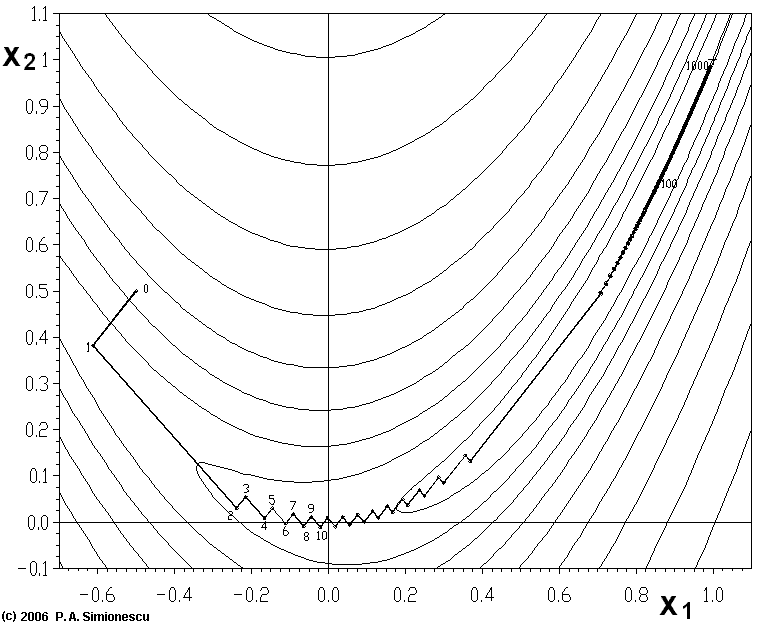## 从梯度下降法、牛顿法到拟牛顿法，浅谈它们的联系与区别

1.梯度下降法

$\space\space\space\space\space\space\space\space\space\space\space\space\space\space\space\space\space\space\space\space\space\space\space\space\space\space\space\space\space\space\space\space\space\space\space\space\space\space\space\space\space\space\space\space\space\space\pmb{x}_{k+1}=\pmb{x}_k-\varepsilon\triangledown_xf(\pmb{x}_k)$2.牛顿法

$\min\limits_{\vec{x}} \left[f(\pmb{x}_0)+(\pmb{x}-\pmb{x}_0)^T\triangledown_xf(\pmb{x}_0)+\frac{1}{2}(\pmb{x}-\pmb{x}_0)^T H(\pmb{x}-\pmb{x}_0)\right]$

$\space\space\space\space\space\space\space\space\space\space\space\space\space\space\space\space\space\space\space\space\space\space\space\space\space\space\space\space\space\space\space\space\space\space\space\space\space\space\space\space\space\space\space\space\space\space\pmb{x}_{k+1}=\pmb{x}_k-H^{-1}\triangledown_xf(\pmb{x}_k)$

3.拟牛顿法

$\space\space\space\space\space\space\space\space\space\space\space\space\space\space\space\space\space\space\space\space\space\space\space\space\space\space\space\space\space\space\space\space\space\space\space\space\space\space\space\space\space\space\space\space\space\space G_{k+1}v_k=G_kv_k+P_kv_k+Q_kv_k$

$\space\space\space\space\space\space\space\space\space\space\space\space\space\space\space\space\space\space\space\space\space\space\space\space\space\space\space\space\space\space\space\space\space\space\space\space\space\space\space\space\space\space\space\space\space\space P_{k}v_k=w_k$
$\space\space\space\space\space\space\space\space\space\space\space\space\space\space\space\space\space\space\space\space\space\space\space\space\space\space\space\space\space\space\space\space\space\space\space\space\space\space\space\space\space\space\space\space\space\space Q_{k}v_k=-G_kv_k$

$\space\space\space\space\space\space\space\space\space\space\space\space\space\space\space\space\space\space\space\space\space\space\space\space\space\space\space\space\space\space\space\space\space\space\space\space\space\space\space\space\space\space\space\space\space\space P_{k}=\frac{w_kw_k^T}{w_k^Tv_k}$
$\space\space\space\space\space\space\space\space\space\space\space\space\space\space\space\space\space\space\space\space\space\space\space\space\space\space\space\space\space\space\space\space\space\space\space\space\space\space\space\space\space\space\space\space\space\space Q_{k}=-\frac{G_kv_kv_k^TG_k}{v_k^TG_kv_k}$

$\space\space\space\space\space\space\space\space\space\space\space\space\space\space\space\space\space\space\space\space\space\space\space\space\space\space\space\space\space\space\space\space\space\space\space\space\space\space\space\space\space\space\space\space\space\space G_{k+1}=G_k+\frac{w_kw_k^T}{w_k^Tv_k}-\frac{G_kv_kv_k^TG_k}{v_k^TG_kv_k}$

$\space\space\space\space\space\space\space\space\space\space\space\space\space\space\space\space\space\space\space\space\space\space\space\space\space\space\space\space\space\space\space\space\space\space\space\space\space\space\space\space\space\space\space\space\space G_{k+1}=P^T\left(I+\frac{Q^Tw_kw_k^TQ^{-1}}{v_k^TH_k^{-1}v_k}-\frac{Pv_kv_k^TP^T}{v_k^TP^TPv_k}\right)P$

$\space\space\space\space\space\space\space\space\space\space\space\space\space\space\space\space\space\space\space\space\space\space\space\space\space\space\space\space\space\space\space\space\space\space\space\space\space\space\space\space\space\space\space\space\space\space\pmb{y}^TO\pmb{y}\ge \pmb{y}^TM\pmb{y}>0$
$\pmb{y}= kPv_k$,则
$\space\space\space\space\space\space\space\space\space\space\space\space\space\space\space\space\space\space\space\space\space\space\space\space\space\space\space\space\space\space\space\space\space\space\space\space\space\space\space\space\space\space\space\space\space\space\pmb{y}^TO\pmb{y}= \pmb{y}^TN\pmb{y}=k^2v_k^TH_k^{-1}v_k>0$

0条评论